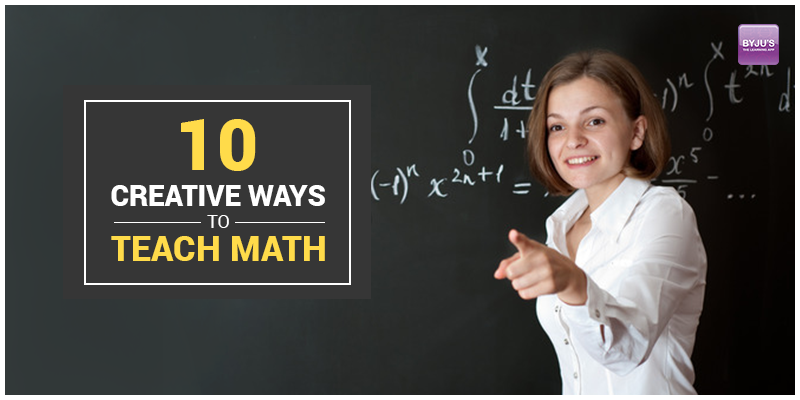Math is one such subject which depends on practice. There are many students who love math while many who do not have a fetish for this subject and finds it quite difficult to solve. This is one such subject where one needs to love it to proceed with the problem. If the candidate does not know the right approach for a specific problem, it becomes tough to execute. To develop a love for math there are many creative ways which the students can follow to learn math. In contrary, the different ways that can be adopted to teach math are:
• Dice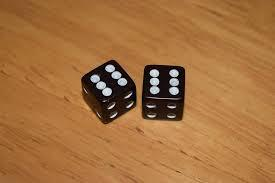One of the best possible way to teach math is by rolling a dice. Dice helps to create their mathematical problems. The fractional problems, multiplication problems or even word problems can be solved with the roll of a dice. This can also be given as home assignment where students can take the problems to solve at home by rolling a dice in the class.
• Math bingo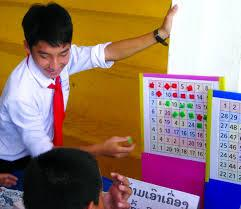This can be a fun loving game to teach math as it is a favourite game for the students in class. Here students have to solve the math sums to know what number is to be eliminated from the sheet. Students will develop an interest through this game to learn math accurately.
• Card gameCard game helps to teach multiplication, addition or even a fractional problem. The different cards make more challenging and exciting for the students to learn math. Even playing a card game helps the candidate to add a bonus in their time of solving a problem. This clearly helps in time management with accuracy which is most essential in acing this subject.
• Sudoku and Kakuro puzzles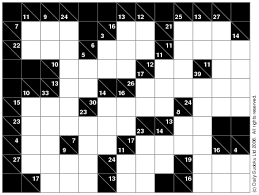Sudoku and Kakuro puzzles are definitely an effective way to enhance their math skill. Kakuro and sudoku puzzles are similar in a way that they come in a grid but the rules are different. This kind of puzzles helps to sharpen the mathematical skill so that it becomes easy to solve whatever numerical problems are assigned to the student.
• Mental math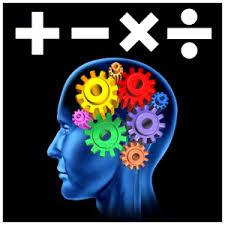There should be classes arranged on mental math where maximum number of the students love to solve. This way is easier to solve and boosts up the confidence of the students to solve other problems. The mental math classes will be interesting for the students and will help the students to develop a fetish for this subject. Mental math also helps to retain the simple addition or multiplication problems in the mind of the students for a longer duration. It is basically the root of the mathematics.
• Math scavenger hunt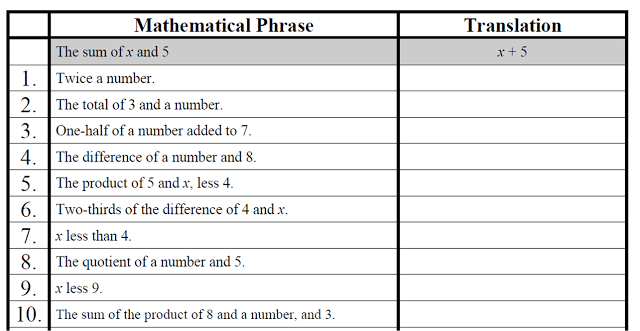This worksheet is to be cut in different questions which help the students to solve in an interesting way. Often students get bored of solving mathematical problems so this scavenger hunt will help students to practice more and not be tired of solving. This can be an effective way to make students practice more and more.
• Math Apps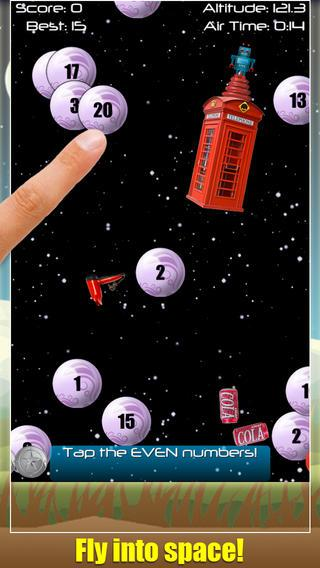Different math apps like matchmaker, Crazy time table and thinking blocks multiplication is best to solve sums even at leisure time. With smart learning classes use of tablets can help them in a better way by installing these math apps on the tablet.
• Using Abacus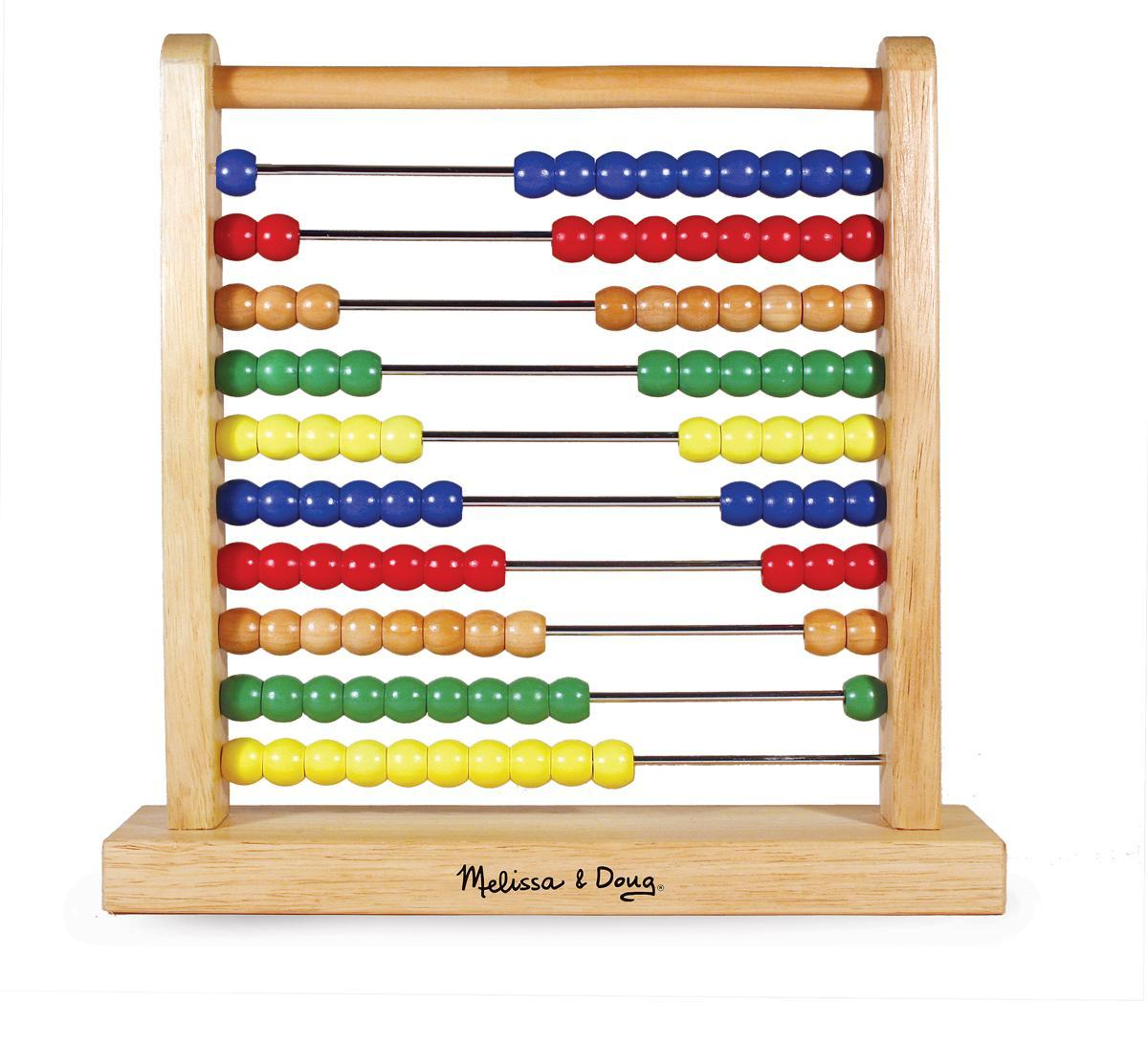Abacus can help the candidates with the simple mathematical calculation by moving the beads. The mental calculation improves efficiently without even using the abacus by practicing more.
• Math lab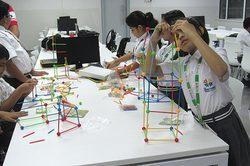Every school should have math lab for the practical implementation of various geometrical shapes. It enhances the geometrical skills of the students for better understanding. The concepts which one visualizes help to retain for a longer duration of time so same is the case for math labs. The practical aspect encourages the student to practice more.
• Math seminars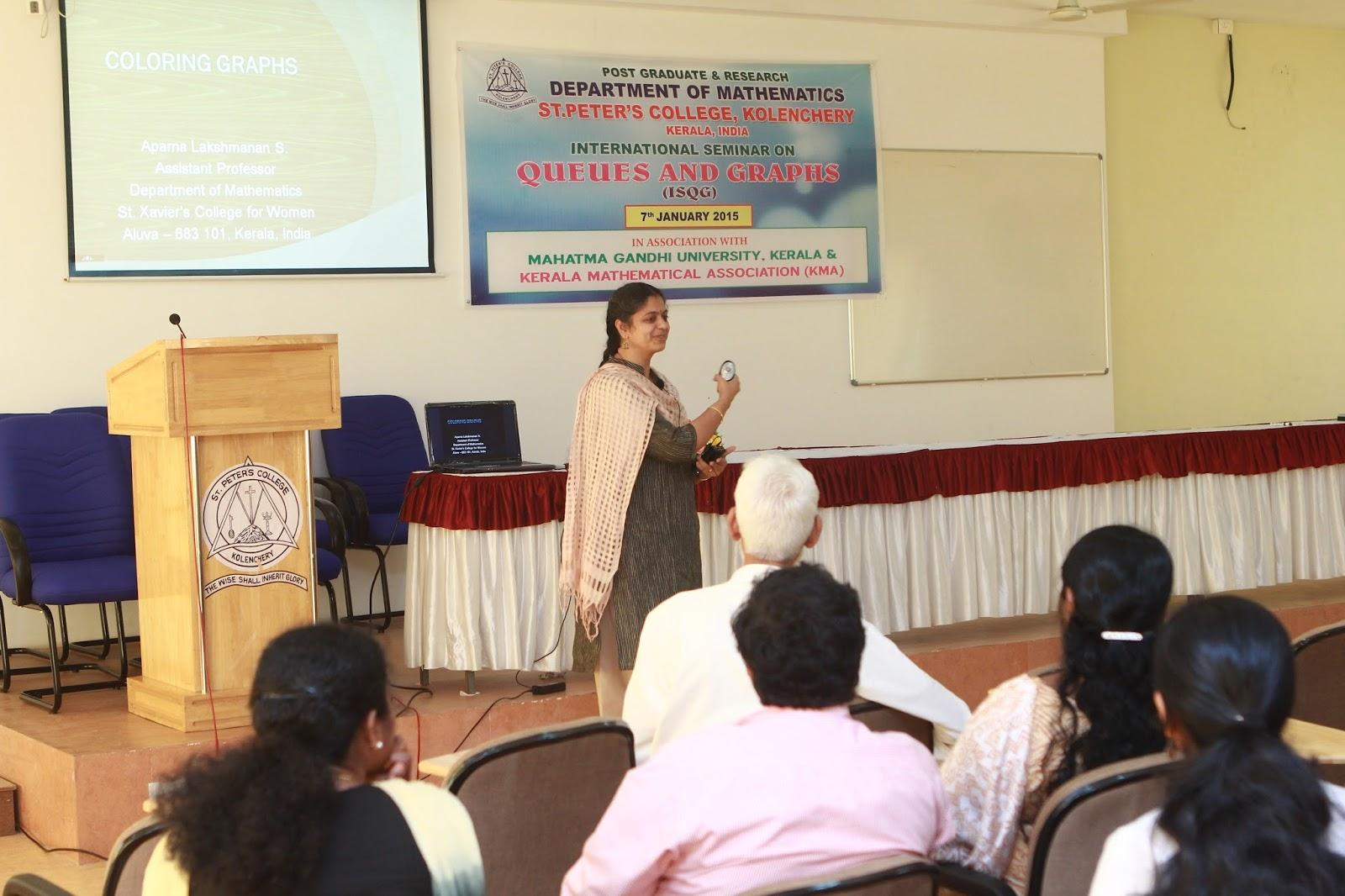Math seminars can be arranged to inspire the students as to how to increase their efficiency and grow more interest to deal with problems. This is one of the best ways to influence students by the speech of the prominent speakers.

The ten creative ways discussed above is interactive and engaging for the students so that math becomes a field of interest where students do not find it difficult to practice. Math is an important subject for board exams. The marks will be reflected in cbse results of the students. The competitive exams like JEE or other exams also need a strong mathematical skill. These ways will surely ensure a good preparation along with practice.